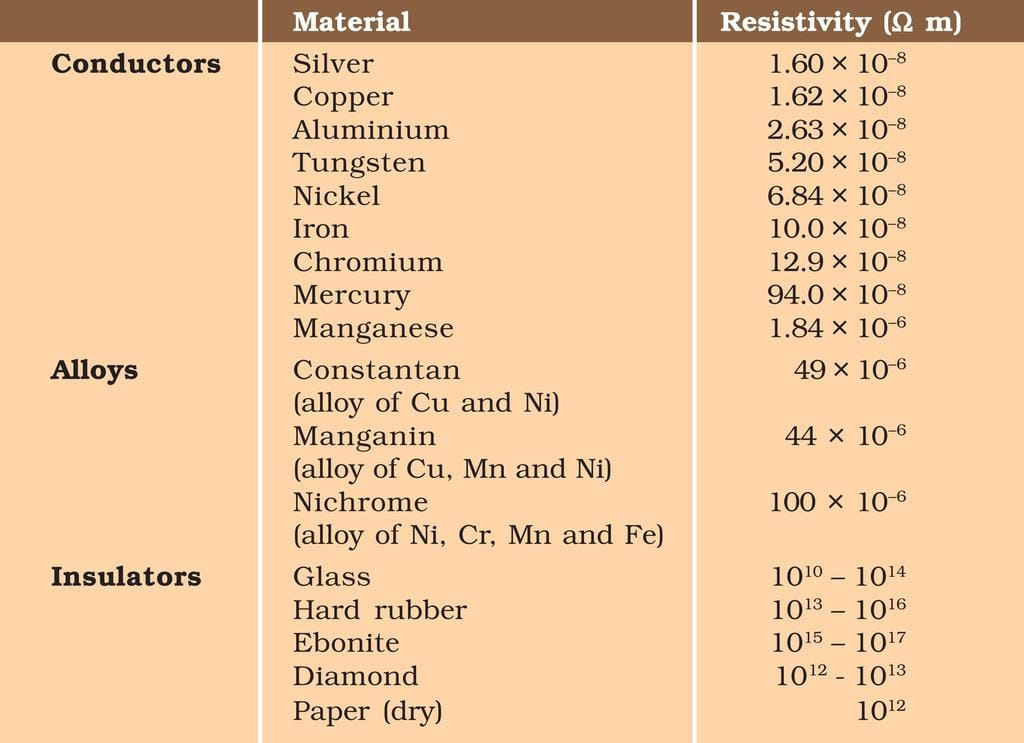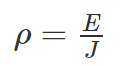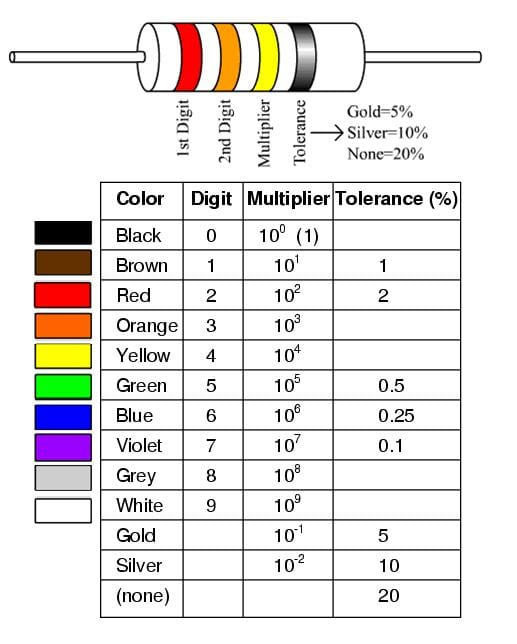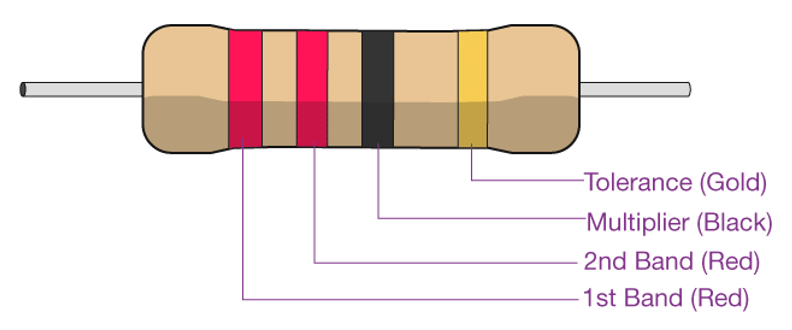NEET  >  Resistivity of Various Materials

# Resistivity of Various Materials | Physics Class 12 - NEET

 1 Crore+ students have signed up on EduRev. Have you?

Resistivity of Materials

We know there are three types of materials; conductors, semiconductors and insulators. Conductors are the materials that can pass electricity through them. In this document, before we learn about electrical resistivity, let us know what is meant by electrical conductivity and its units.
Electrical Conductivity is an intrinsic property of a material which is defined as the measure of the amount of electrical current a material can carry. Electrical conductivity is also known as specific conductance, and the SI unit is Siemens per meter (S/m). It is also defined as the ratio of the current density to the electric field strength. It is represented by the Greek letter σ.Electrical conductivity is a measure of how readily a material transmits an electrical current.

What is Electrical Resistivity?

Electrical resistivity is the reciprocal of electrical conductivity. It is the measure of the ability of a material to oppose the flow of current.

• Metals are good conductors of electricity. Hence, they have low resistivity.
• The insulators like rubber, glass, graphite, plastics, etc. have very high resistivity when compared to the metallic conductors.
• The third type is the semiconductor which comes in between the conductors and insulators. Their resistivity decreases with the increase in temperature and is also affected by the presence of impurities in them.

Resistivity of Different Materials

The table below lists the electrical resistivity of several conductors, semiconductors, and insulators.Resistivity Formula

Materials having electric field and current density will have the following resistivity formula:Where,

• ρ is the resistivity of the material in Ω.m
• E is the magnitude of the electric field in V.m-1
• J is the magnitude of current density in A.m-2

Conductors with a uniform cross-section and uniform flow of electric current will have the following resistivity formula:Where,

• ρ is the resistivity of the material in Ω.m
• R is the electrical resistance of uniform cross-sectional material in Ω
• l is the length of a piece of material in m
• A is the cross-sectional area of the material in m2

Resistivity Unit

Following is the unit of resistivity:

 CGS unit Ω.cm SI unit Ω.m

Dimension of resistivity M1L3T-3A-2

Factors determining Resistivity of Materials

Following are the factors that determine the resistivity of materials:

• Nature of material
• Temperature

Resistor Colour-Coding

Resistors are used in electrical circuits to control or reduce the flow of current in the circuit. Their resistance is indicated by using electronic color codes. Different color bands or rings are marked on these resistors for different values of resistance.Resistor Colour Code and
Resistor Tolerance explained
Highlights of the resistor color-coding are as follows:

• It usually contains four bands.
• The first band is indicative of the first significant figure of the resistance.
• The second band is the second significant figure. (At times there is a third band to have more precision and hence, they are 5 band resistors.)
• The third band is the decimal multiplier.
• The fourth band is indicative of the tolerance (in percentage) that the resistor can withstand the indicated values.
• In the absence of the fourth band, a default tolerance of 20% is taken.

Let us take an example to understand resistor color coding.• The resistor has four color bands.
• The first band is red. From the table, we know that red stands for the significant digit 2.
• The second is red too. This is indicative of the second significant digit 2.
• The third band is a multiplier and is black. For black, the multiplier is 100 or 1.
• The fourth band is gold which indicates a tolerance of ±5%.
• Hence, the resistor has a resistance of 22 x 1 Ω with ±5% tolerance.

1. What is the SI unit of electrical resistivity?
SI unit of electrical resistivity is the ohm-meter.

2. What is the relationship between electrical conductivity and electrical resistivity?
The relationship between electrical conductivity and electrical resistivity is
σ = 1/ρ

3. Is rubber a good conductor of electricity?
Rubber cannot pass electricity, hence is not a conductor.

4. Explain the conductance of a semiconductor?
The conducting capacity of semiconductors lies between a conductor and an insulator.

5. Give some examples of good conductors?

• Gold
• Silver
• Copper
• Aluminum
• Zinc
• Brass
The document Resistivity of Various Materials | Physics Class 12 - NEET is a part of the NEET Course Physics Class 12.
All you need of NEET at this link: NEET

## Physics Class 12

157 videos|452 docs|213 tests

## Physics Class 12

157 videos|452 docs|213 tests

### How to Prepare for NEET

Read our guide to prepare for NEET which is created by Toppers & the best Teachers

Track your progress, build streaks, highlight & save important lessons and more!(Scan QR code)

,

,

,

,

,

,

,

,

,

,

,

,

,

,

,

,

,

,

,

,

,

;